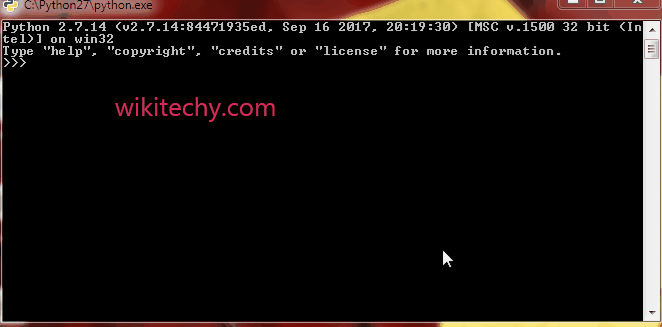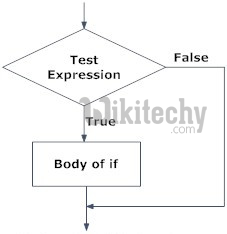# python tutorial - Python if | Python if Statement - learn python - python programming

• The if statement in python is same as c language which is used test a condition.
• If condition is true, statement of if block is executed otherwise it is skipped.Learn Python - Python tutorial - python if - Python examples - Python programs

## Python if Statement Flowchart## Syntax for If Statement:

• Here, the program evaluates the test expression and will execute statement(s) only if the text expression is True.
• If the text expression is False, the statement(s) is not executed.
• In Python, the body of the if statement is indicated by the indentation. Body starts with an indentation and the first unindented line marks the end.
• Python interprets non-zero values as True. None and 0 are interpreted as False.

## Example: Python if Statement

• If the number is positive, we print an appropriate message
• When you run the program, the output will be:
• In the above example, num > 0 is the test expression.
• The body of if is executed only if this evaluates to True.
• When variable num is equal to 3, test expression is true and body inside body of if is executed.
• If variable num is equal to -1, test expression is false and body inside body of if is skipped.
• The print() statement falls outside of the if block (unindented). Hence, it is executed regardless of the test expression.

Wikitechy tutorial site provides you all the learn python , python code , python script , python windows , python mysql , django python , python django , python compiler , jython , python interpreter , what is python , python join , python input , python modules , python editor , python examples , python pdf , python documentation